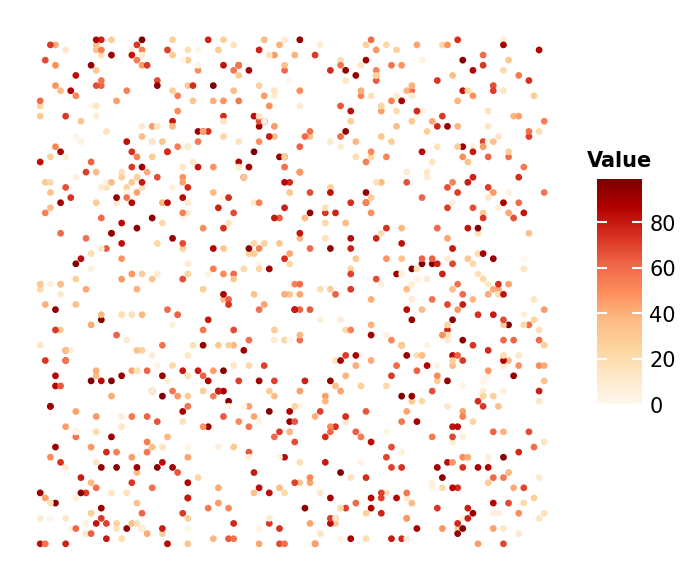# Point map Example (Continuous data)#

Here shows how to draw the point map

```import numpy as np
import milkviz as mv
```

## First let’s create some random data#

```np.random.seed(0)
xy = np.random.randint(0, 100, (1000, 2))
values = np.random.randint(0, 100, 1000)
```

## Create the cell map#

```mv.point_map(xy, values=values, cbar_kw={"title": "Value"})
``````<AxesSubplot: >
```

Total running time of the script: ( 0 minutes 1.045 seconds)

Gallery generated by Sphinx-Gallery